The Power Factor and Complex Power
How to alter the Power Factor of a Load
and the purpose in doing so.

 Outline: What is the Power Factor and Apparent Power? Altering the Power Factor of a Load Complex Power

What is the Power Factor and Apparent Power?

Recall the equation for average power:    ½ VmIm cos (θ)
The Power Factor is simply cos(θ):    pf = cos (θ)

Therefore we can rewrite average power as:    ½ VmIm (pf)

Recall that θ is the angle of the impedance Z

In a purely resistive load, θ=0, therefore the pf=1.

In a purely reactive load (all capacitance or all inductance), θ=±90°, therefore the pf=0.

Note that the angle of Z must be between +90° and -90° because if there is any resistance,
resistance can not be negative. Only reactance can be negative, as is the case with a capacitor.
Since pf = cos(θ), then the pf will always be some number between 0 and 1.
The power factor is never negative because resistance is never negative.

If the pf = ½, how do you know if θ= +60° or -60°?   You don't unless
there is some way of specifying that the reactance is either inductive or capacitive.

The table below shows how we make this distinction.

 LAGGING Any angle in this quadrant had a net positive reactance and therefore has mostly inductance: Power Factors for loads with impedances in this quadrant are said to be lagging because for these loads the current lags the voltage. So if the pf = ½  lagging, then we know the angle of Z is + 60°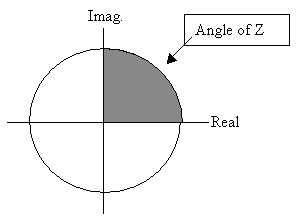LEADING Any angle in this quadrant had a net negative reactance and therefore has mostly capacitance: Power Factors for loads with impedances in this quadrant are said to be leading because for these loads the current leads the voltage. So if the pf = ½  leading, then we know the angle of Z is - 60°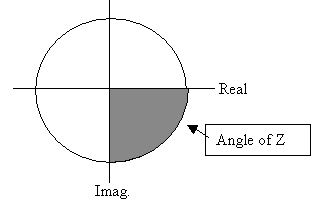If one states that the pf= ½ or pf = 0.30 without stating 'leading' or 'lagging' then there is incomplete information. The power factor must state either leading or lagging.

Apparent Power is simply:   Papparent= VRMS IRMS

Therefore we can express Average Power as:    Paverage= Papparent × pf

We can also say:    pf = Paverage/Papparent

In other words, the power factor is the percentage of Apparent Power
that is actually being absorbed by the load.

Summary

We now have additional equations for calculating Average Power:

pf = cos(θ) = real(Z)/|Z|

Paverage = VRMS IRMS × pf
Paverage = Papparent × pf

After clicking on the following link enter 12-1 for the problem and 1 for the step:
Study Problem 12-1

Top of Page

Altering the Power Factor of a Load

First what is the purpose of altering the Power Factor of a Load?

The Power Factor affects the cost of transmitting power.
Utility companies want to minimize the cost of transmitting power in order to
maximize their earnings.

Note that:    Paverage = VRMS IRMS × pf

Rearranging this equation gives:    IRMS = Paverage/(VRMS × pf)

The power the utility company needs to generate is Pg:
Pg = Power needed by Customers + Power Losses on the Transmission Lines

Power needed by Customers is determined by customer demand, therefore the utility company has no influence of this.

However Power Losses on the Transmission Lines is something the utility company can try to minimimze.
It is also something the utility company can not charge for, thus transmission line losses represent lost earnings.

Power Losses on the Transmission Lines = (IRMS)2 × (Resistance of Transmission Lines)

Therefore how does the utility company minimimze the following equation:
IRMS = Paverage/(VRMS × pf)

VRMS is fixed. The U.S. operates on fixed voltages, such as 120V or 220V etc.
Paverage is fixed. Customer demand determines how much customers use.

However the Power Factor (pf) is not fixed.
pf is something that can be maximized by users at the utility company's request.

The closer the pf is to one, the lower IRMS is.

How to change the pf of a Load:
 Here is a load. Think if this load as a large utility customer, such as Paramount's Great America: If Great America's pf is not close to one, then the utility company has to generate a greater IRMS thus incurring greater transmission line losses. Great America only has to pay for the average power they use, not the losses on the transmission lines.Here is the same load with another load in parallel with it: The effective load ZT can now have a power factor equal to one. At the same time no change has been made to the Great America load - no retooling. Thus Great America still pays for same amount of Average Power as before. We will select Z1 such that: Z1 does NOT absorb any power. Meaning Z1 is purely reactive. ZT has a power factor as close to one as possible.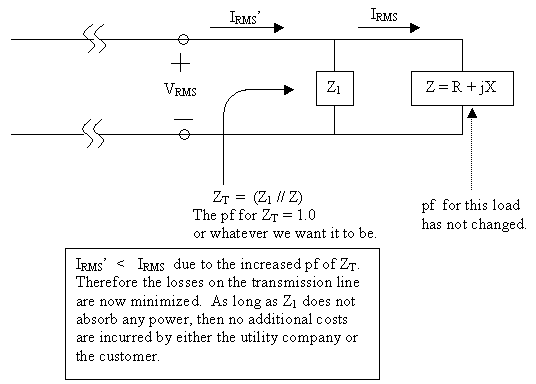So how does one find Z1?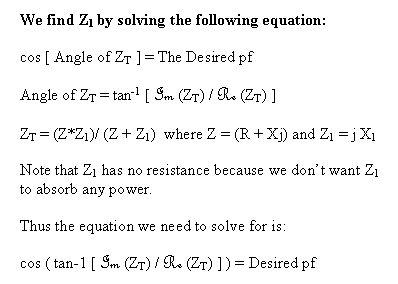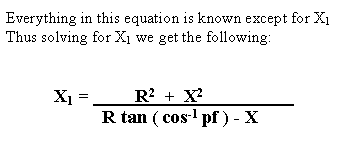Note that if the desired pf is lagging, then (cos-1 pf) is a positive angle.
Similarly if the desired pf is leading, then (cos-1 pf) is a negative angle.

## Study Problems

After clicking on the following link enter 12-2 for the problem and 1 for the step:
Study Problem 12-2

Top of Page

Complex Power

Complex Power is: VRMS IRMS e

Recall that Paverage = VRMS IRMS  cos(θ) = Real [VRMS IRMS e]

Therefore Real[ Complex Power ] = Paverage

The imaginary part of Complex Power is called Reactive Power.

The symbol for Reactive Power is Q:

Q = Imaginary[ Complex Power ] = VRMS IRMS  sin(θ)

The symbol for Complex Power is S:

Therefore expressing Complex Power in rectangular form:    S = Paverage + jQ

Expressing Complex Power in polar form:    S = VRMS IRMS /θ

Summary

Complex Power is a complex number.
It can be shown graphically as:θ is still the angle of the impedance.

After clicking on the following link enter 12-3 for the problem and 1 for the step:
Study Problem 12-3

Top of Page
Back To Index